# Activity: Commutative, Associative and Distributive

Learn the difference between Commutative, Associative and Distributive Laws by creating:

Comic Book Super HeroesYou will need:

• Coloring pencils
• Paper
• Imagination!

## Activity 1

Step 1: The "Commutative Laws" say you can swap numbers over and still get the same answer ...

a + b  =  b + a

### Example:... or when you multiply:

a × b  =  b × a

### Example: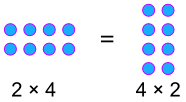Step 2: Does order of numbers matter when multiplying or adding?

Step 3: Using this information, try to draw a super hero whose super powers incorporates the fact that order doesn't matter.

Your super hero could be a man, a woman, a dog, a robot, anything!

Step 4: Label your super hero Commutative (Man/Woman/Dog/Whatever)

## Activity 2:

Step 1: The "Associative Laws" say that it doesn't matter how you group the numbers (i.e. which you calculate first) ...

(a + b) + c  =  a + (b + c)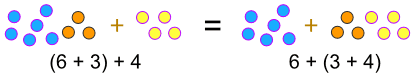... or when you multiply:

(a × b) × c  =  a × (b × c)

###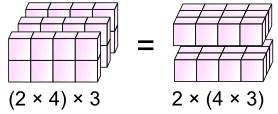Step 2: Do the way we group numbers matter when multiplying or adding?

Step 3: Using this information, try to draw a super hero whose super powers incorporates the fact that the way we group numbers doesn't matter

Step 4: Label your super hero Associative (Girl/Boy/Dog/Whatever)

## Activity 3

Step 1: The "Distributive Law" says:

a × (b + c)  =  a × b  +  a × c

This is what it lets you do: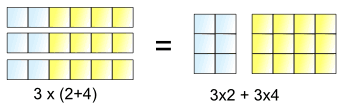3 lots of (2+4) is the same as 3 lots of 2 plus 3 lots of 4

Step 2: Though they kind of look the same, what's different between Associative and Distributive. Notice that one uses multiplication and addition, but the other uses either multiplication or addition. What else can you notice

Step 3: Using this information, try to draw a super hero whose super powers are distributive

Step 4: Label your super hero Distributive (Woman/Man/Hamster/Whatever)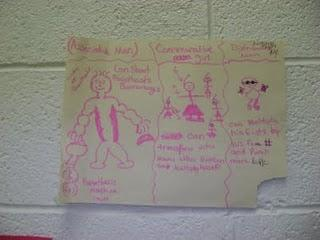Put your illustrations on the wall!

Activity courtesy of Hands On Math